JEE  >  JEE Main Mock - 11 (Previous Pattern)

# JEE Main Mock - 11 (Previous Pattern)

Test Description

## 90 Questions MCQ Test Mock Test Series for JEE Main & Advanced | JEE Main Mock - 11 (Previous Pattern)

JEE Main Mock - 11 (Previous Pattern) for JEE 2022 is part of Mock Test Series for JEE Main & Advanced preparation. The JEE Main Mock - 11 (Previous Pattern) questions and answers have been prepared according to the JEE exam syllabus.The JEE Main Mock - 11 (Previous Pattern) MCQs are made for JEE 2022 Exam. Find important definitions, questions, notes, meanings, examples, exercises, MCQs and online tests for JEE Main Mock - 11 (Previous Pattern) below.
Solutions of JEE Main Mock - 11 (Previous Pattern) questions in English are available as part of our Mock Test Series for JEE Main & Advanced for JEE & JEE Main Mock - 11 (Previous Pattern) solutions in Hindi for Mock Test Series for JEE Main & Advanced course. Download more important topics, notes, lectures and mock test series for JEE Exam by signing up for free. Attempt JEE Main Mock - 11 (Previous Pattern) | 90 questions in 180 minutes | Mock test for JEE preparation | Free important questions MCQ to study Mock Test Series for JEE Main & Advanced for JEE Exam | Download free PDF with solutions
 1 Crore+ students have signed up on EduRev. Have you?
JEE Main Mock - 11 (Previous Pattern) - Question 1

### Area enclosed by the curve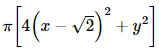= 8 is

Detailed Solution for JEE Main Mock - 11 (Previous Pattern) - Question 1

The given equation can be rewritten as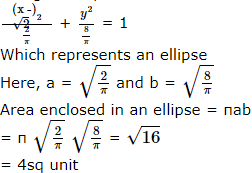JEE Main Mock - 11 (Previous Pattern) - Question 2

### If n ∈ N then n3+2n is divisible by

JEE Main Mock - 11 (Previous Pattern) - Question 3

### x=7 touches the circle x2 + y2 - 4x - 6y - 12 = 0, then the co-ordinates of the point of contact are

JEE Main Mock - 11 (Previous Pattern) - Question 4

If ω is a cube root of unity ,then
(1 - ω + ω2)5 + (1 + ω - ω2) is equal to

JEE Main Mock - 11 (Previous Pattern) - Question 5

If z = -2/(1 + √3i),then the value of arg (z) is

JEE Main Mock - 11 (Previous Pattern) - Question 6

The solution of the differential equation cos x sin y dx + sin x cos y dy =0 is

JEE Main Mock - 11 (Previous Pattern) - Question 7

If m and n are integers, then what is the value of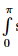sin m x sin n x d x . If m ≠ n

Detailed Solution for JEE Main Mock - 11 (Previous Pattern) - Question 7

Since sin mx, sin nx is an odd function if m ≠ n,  then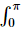sin mx . sin nx dx = 0

JEE Main Mock - 11 (Previous Pattern) - Question 8

The value of f(0) so that f (x)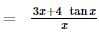is continuous at x = 0 is

JEE Main Mock - 11 (Previous Pattern) - Question 9

The solution of the equation xdy-ydx=0 is

JEE Main Mock - 11 (Previous Pattern) - Question 10

If y=sec⁻1[(x+1)/(x-1)]+sin⁻1[(x-1)/(x+1)], (dy/dx)=

JEE Main Mock - 11 (Previous Pattern) - Question 11

Tangents are drawn from a point P(3,4) to the ellipse (x2/9) + (y2/16) = 1 touching the ellipse in the points Q and R. The angle between PQ and PR is

JEE Main Mock - 11 (Previous Pattern) - Question 12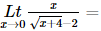JEE Main Mock - 11 (Previous Pattern) - Question 13

Which statement is logically equivalent to "If Yoda cannot use a lightsaber, then he cannot help Luke win the battle."

Detailed Solution for JEE Main Mock - 11 (Previous Pattern) - Question 13

A statement is logically equivalent to its contrapositive. To form the contrapositive, switch the "If" and "then" sections of the statement AND insert "NOTs" into each section. Notice in this situation, that inserting "NOTs" turns the thoughts positive (you are negating negative thoughts).

JEE Main Mock - 11 (Previous Pattern) - Question 14

In the following question, a Statement of Assertion (A) is given followed by a corresponding Reason (R) just below it. Read the Statements carefully and mark the correct answer-
Assertion(A): Let f(x) and g(x) be two polynomials all of whose coefficients are rational numbers . Suppose f(x) and g(x) have a common irrational root, then g(x) = r f(x) for some rational number.
Reason(R): The common root of the equations ax2 + bx + c = 0 and a'x2 + b'x + c' = 0 is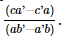JEE Main Mock - 11 (Previous Pattern) - Question 15

In the following question, a Statement of Assertion (A) is given followed by a corresponding Reason (R) just below it. Read the Statements carefully and mark the correct answer-
Assertion(A):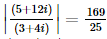Reason(R):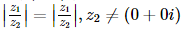JEE Main Mock - 11 (Previous Pattern) - Question 16
In the following question, a Statement of Assertion (A) is given followed by a corresponding Reason (R) just below it. Read the Statements carefully and mark the correct answer-
Assertion(A): y = 0 is the singular solution of the equation 9yp2 + 4 = 0.
Reason(R): y = 0 occurs both in the p-discriminant , and c-discriminant obtained from the general solution y3 + (x+c)2 = 0 of the equation 9yp2 + 4 = 0.
JEE Main Mock - 11 (Previous Pattern) - Question 17

If each element of a 3 x 3 matrix is multiplie by 3, then the determinant of the newly formed matrix is

JEE Main Mock - 11 (Previous Pattern) - Question 18
The maximum value of [(logx)/x] is
JEE Main Mock - 11 (Previous Pattern) - Question 19
Sum of the deviations about mean is:
JEE Main Mock - 11 (Previous Pattern) - Question 20

In the following question, a Statement of Assertion (A) is given followed by a corresponding Reason (R) just below it. Read the Statements carefully and mark the correct answer-
Assertion(A):α1234 are roots of 2x4 - 2x3 + 9x2 - x + 12 = 0, then the value of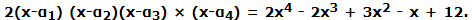is 21.
Reason(R): 2(x-α1) (x-α2)(x-α3) x  (x-α4) = 2x4 - 2x3 + 3x2 - x + 12. Put x = 3 i on both sides and take modulus of both sides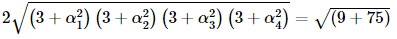JEE Main Mock - 11 (Previous Pattern) - Question 21

The line 4x + 6y + 9 = 0 touches the parabola y2 = 4 x at the point

JEE Main Mock - 11 (Previous Pattern) - Question 22
If nCr+nCr+1=n+1Cx, then x=
JEE Main Mock - 11 (Previous Pattern) - Question 23
If the sum of series 2 + 5 + 8 + .... upto n terms is 60100, then n
JEE Main Mock - 11 (Previous Pattern) - Question 24
A bag contains 5 brown and 4 white socks. A man pulls out two socks. The probability that they are of the same colour is
JEE Main Mock - 11 (Previous Pattern) - Question 25

If A = [(x,y) : x2 + y2 = 25] and B = [(x,y) : x2 + 9y2 = 144] and A ∩ B contains

JEE Main Mock - 11 (Previous Pattern) - Question 26

A random variable X has the following distribution
x                  1    2   3  4
P ( X = x)     c  2c 3c 4c
The value of c is

JEE Main Mock - 11 (Previous Pattern) - Question 27

The plane XOZ divides the join of (1,-1,5) and (2,3,4) in the ratio λ:1,then λ is

Detailed Solution for JEE Main Mock - 11 (Previous Pattern) - Question 27

Since the plane XOZ divides the join of (1, -1, 5) and (2,3,4) in the ratio λ:1.
S o . Y = 0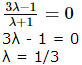JEE Main Mock - 11 (Previous Pattern) - Question 28
If sin θ₁ + sin θ₂ + sin θ₃=3, then cosθ₁ + cosθ₂ + cos θ₃
JEE Main Mock - 11 (Previous Pattern) - Question 29

The angle between the vectors
î - ĵ + k̂ and î + 2ĵ + k̂ is

JEE Main Mock - 11 (Previous Pattern) - Question 30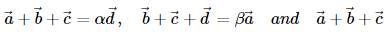are non− coplanar, then the sum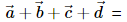JEE Main Mock - 11 (Previous Pattern) - Question 31

Alcohol fermentation is brought about by the action of

JEE Main Mock - 11 (Previous Pattern) - Question 32

Which of the following radicals will not be precipitated by passing H₂S in concentrated acid solution?

JEE Main Mock - 11 (Previous Pattern) - Question 33

The wavelength of light having wave number 4000cm-1 is

JEE Main Mock - 11 (Previous Pattern) - Question 34

IUPAC name of CH₃-O-C₂H₅ is

JEE Main Mock - 11 (Previous Pattern) - Question 35

Purine derivative among the following bases is

JEE Main Mock - 11 (Previous Pattern) - Question 36

Amongst the following the molecule that is linear is

JEE Main Mock - 11 (Previous Pattern) - Question 37

The correct order of hybridisation of the central room atom in the following species, NH₃, [PtCl₄]2⁻, PCl₅ and BCl₃ is respectively

JEE Main Mock - 11 (Previous Pattern) - Question 38

The types of bonds present in CuSO₄.5H₂O are

JEE Main Mock - 11 (Previous Pattern) - Question 39

Latent heat of vaporisation of a liquid at 500 K and 1 atm pressure is 10.00 Kcal/mol. What will be the change in internal energy (ΔU) of 3 mol of liquid at same temperature ?

Detailed Solution for JEE Main Mock - 11 (Previous Pattern) - Question 39

Vaporization of 3 moles of H2O vapors is: 3H2O(l) → 3H2O(g)
Δn = 3 - 0 = 3
Therefore,
ΔU = ΔH - ΔnRT
= (3 x 10) - 3(0.002)(500) = 27
change in internal energy is:
ΔU = 27 Kcal

JEE Main Mock - 11 (Previous Pattern) - Question 40

A liquid is in equilibrium with its vapour at its boiling point. The molecules in these two phases will have equal

JEE Main Mock - 11 (Previous Pattern) - Question 41

If 75% of first order reaction is completed in 32 min, then 50% of the reaction would be completed in

JEE Main Mock - 11 (Previous Pattern) - Question 42

The bond dissociation energies of H₂,Cl₂ and HCl are 104, 58 and 103 kcal respectively. The enthalpy of formation of HCl gas will be

JEE Main Mock - 11 (Previous Pattern) - Question 43

The enthalpy and entropy change for a chemical reaction are -2.5 X 103 J-mol⁻1 and 7.4 J-K⁻1-mol⁻1 respectively. The reaction at 298 K is

JEE Main Mock - 11 (Previous Pattern) - Question 44

In an endothermic reaction, the value of ∆H is

JEE Main Mock - 11 (Previous Pattern) - Question 45

IUPAC name of CH₂ = CH - CH is

JEE Main Mock - 11 (Previous Pattern) - Question 46

Eka-aluminium and eka-silicon are known as

Detailed Solution for JEE Main Mock - 11 (Previous Pattern) - Question 46

Gallium and Germanium were unknown at the time when Mendeleev's periodic table was formulated. But Mendeleev's predicted the properties of these elements and named them eka-aluminium and eka-silicon. Later when these elements were discovered, their properties were found to be similar to those of aluminium and silicon.

JEE Main Mock - 11 (Previous Pattern) - Question 47

The atomic radius of elements of which of these would be nearly same

Detailed Solution for JEE Main Mock - 11 (Previous Pattern) - Question 47

The atomic radii of the d- block elements of a given series decrease with increase in atomic number but this decrease becomes small after midway. The decrease in atomic radii in the beginning, is due to an increase in nuclear charge from member to member which tends to reduce the size. At the same time, the addition of extra electrons to (n-1)d orbitals also provides the screening effect. As the number of d-electrons increases, the screening effect increases. Thus, there are operating two effects namely screening effect and nuclear charge effect which oppose each other. In the midway onwards of the series both these effects become nearly equal and thus, there is no change in atomic radii inspite of the fact that atomic number increases gradually.

JEE Main Mock - 11 (Previous Pattern) - Question 48

Low spin octahedral complexes are maximum distorted in which of the following state?

Detailed Solution for JEE Main Mock - 11 (Previous Pattern) - Question 48

whenever eg orbitals unsymmetrical, i.e., contain 1,2 or 3 electons in low spin complex strong distortion occurs leading to tetrgonal or square planer complexes. Since the direction of the eg orbitals of the central metals ion correspond with the directions of the ligands disposed octahedrally around the metal ion, the octahedral arrangement of the ligands is likely to be more severely distorted by the dissymmetry of the eg orbitals than that of the t2g orbitals.

JEE Main Mock - 11 (Previous Pattern) - Question 49

Which of the following factors may be regarded as the main cause of lanthanide contraction?

JEE Main Mock - 11 (Previous Pattern) - Question 50

In alkaline medium ClO₂ oxidises H₂O₂ to O₂ and itself gets reduced to Cl⁻.How many moles of H₂O₂ are oxidised by 1 mole of ClO₂ ?

JEE Main Mock - 11 (Previous Pattern) - Question 51

The electrode potentials of half-cell reactions are: Zn → Zn2⁺ + 2e⁻ ; E = -0.76 V and Fe → Fe2⁺ + 2e⁻ ; E=0.41 V. The e.m.f. of the cell Fe2⁺ + Zn → Zn2⁺ + Fe is

JEE Main Mock - 11 (Previous Pattern) - Question 52

50 mL of hydrogen diffues through a small hole from a vessel in 20 min .The time needed for 40 mL of oxygen to diffuses out is

JEE Main Mock - 11 (Previous Pattern) - Question 53

Basicity of orthophosphoric acid is

JEE Main Mock - 11 (Previous Pattern) - Question 54

Which one of the following statements ,if any ,regarding hydrogen peroxide is false ?

JEE Main Mock - 11 (Previous Pattern) - Question 55

Reaction C₂H₅I + C₅H₁₁I + 2Na → C₂H₅ - C₅H₁₁ + 2NaI is called

JEE Main Mock - 11 (Previous Pattern) - Question 56

When wine is put in air, it becomes sour due to

JEE Main Mock - 11 (Previous Pattern) - Question 57

Arrange the following halides in the decreasing order of SN1 reactivity.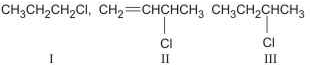JEE Main Mock - 11 (Previous Pattern) - Question 58

In order to get Bakelite from phenol which of the following reagents is required?

JEE Main Mock - 11 (Previous Pattern) - Question 59

Arrange these compounds in order of increasing SN1 reaction rate :

JEE Main Mock - 11 (Previous Pattern) - Question 60

The Fisher projections shown to the right are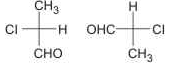JEE Main Mock - 11 (Previous Pattern) - Question 61

The particles which can be added to the nucleus of an atom without changing its chemical properties are called

JEE Main Mock - 11 (Previous Pattern) - Question 62

The half-life of radium is about 1600 years. If 100 g of radium is existing now, then 25 g will remain unchanged after

JEE Main Mock - 11 (Previous Pattern) - Question 63

Two wires of equal diameters of resistivities ρ1 and ρ2 and lengths X1 and X2 respectively are joined in series. The equivalent resistivity of the combination is

JEE Main Mock - 11 (Previous Pattern) - Question 64

Resistance of a coli is 4.2 Ω at 100ºC and the temperature coefficient of resistance of its material is 0.004/ºC. Then its resistance at 0ºC is

JEE Main Mock - 11 (Previous Pattern) - Question 65

In a discharge tube at 0.02 mm, there is formation of

JEE Main Mock - 11 (Previous Pattern) - Question 66

The approximated wavelength of a photon of energy 2.48eV is

JEE Main Mock - 11 (Previous Pattern) - Question 67

According to Maxwell's hypothesis, a changing electric field gives rise to

JEE Main Mock - 11 (Previous Pattern) - Question 68

Two charged spheres separated by a distance 'd' exert some force (F) on each other. If they are immersed in a liquid of dielectric constant 4, then what is the force exerted, if all other conditions are same?

Detailed Solution for JEE Main Mock - 11 (Previous Pattern) - Question 68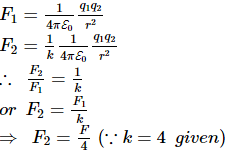JEE Main Mock - 11 (Previous Pattern) - Question 69

The orbital velocity a satellite near the surface of the earth is 7 km-s⁻1. If radius of the orbit is 4 times the radius of earth, then its orbital velocity will be

JEE Main Mock - 11 (Previous Pattern) - Question 70

Two capacitors of capacitances 3 μF and 6 μF are charged to a potential of 12 V each. They are now connected to each other, with the positive plate of each joined to the negative plate of the other. The potential difference across each capacitor will be

JEE Main Mock - 11 (Previous Pattern) - Question 71

If the value of g at the surface of the earth is 9.8 m/sec2, then the value of g at a place 480 km above the surface of the earth will be

JEE Main Mock - 11 (Previous Pattern) - Question 72

On increasing the temperature of a substance gradually, which of the following colours will be noticed by you

JEE Main Mock - 11 (Previous Pattern) - Question 73

When a bus suddenly takes a bend, the passengers are thrown in the outward direction, due to

JEE Main Mock - 11 (Previous Pattern) - Question 74

Heat travels through vacuum by

JEE Main Mock - 11 (Previous Pattern) - Question 75

A moving charge will gain energy due to the application of

JEE Main Mock - 11 (Previous Pattern) - Question 76

For a moving body at any instant of time

JEE Main Mock - 11 (Previous Pattern) - Question 77

A particle is viberating in a simple harmonic motion with an amplitude of 4 cm. At what displacement from the equilibrium position, is its energy half potential and kinetic ?

JEE Main Mock - 11 (Previous Pattern) - Question 78

In the following question, a Statement of Assertion (A) is given followed by a corresponding Reason (R) just below it. Read the Statements carefully and mark the correct answer-
Assertion(A): The combination Y = sinωt + cos 2ωt is not a simple harmonic function even though it is periodic.
Reason(R): All periodic functions satisfy the relation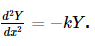JEE Main Mock - 11 (Previous Pattern) - Question 79

In the following question, a Statement of Assertion (A) is given followed by a corresponding Reason (R) just below it. Read the Statements carefully and mark the correct answer-
Assertion(A): The equation y = x + t cannot be true, where x, y are distance and t is time.
Reason(R) : Quantities with different dimensions cannot be added.

JEE Main Mock - 11 (Previous Pattern) - Question 80

In the following question, a Statement of Assertion (A) is given followed by a corresponding Reason (R) just below it. Read the Statements carefully and mark the correct answer-
Assertion(A): P-T graphs of all gases at low density meet at only one point called absolute zero.
Reason(R): Absolute zero is less than 0ºC in a celsius scale.

JEE Main Mock - 11 (Previous Pattern) - Question 81

According to kinetic theory of gases the absolute temperature of a gas is directly related to the average of

JEE Main Mock - 11 (Previous Pattern) - Question 82

A glass rod is dipped in mercury and withdrawn out, the mercury does not wet the rod because

JEE Main Mock - 11 (Previous Pattern) - Question 83

While viewing a distant object with a telescope, suddenly a housefly sits on objective lens. The correct statement is that

JEE Main Mock - 11 (Previous Pattern) - Question 84

To move a body in a circle, which of the following force is needed?

JEE Main Mock - 11 (Previous Pattern) - Question 85

A long spring when stretched by 'x' cm has a potantial energy V. On increasing the stretching to 'nx' cm the potential energy stroed in the spring will be

JEE Main Mock - 11 (Previous Pattern) - Question 86

To hear an echo, the reflecting surface must be at a minimum distance of

Detailed Solution for JEE Main Mock - 11 (Previous Pattern) - Question 86

To hear the echo distinctly, the time gap between the two sounds must be 0.1 s.
Let the distance between the listener and the reflecting surface be d
The total distance travelled by the sound = 2d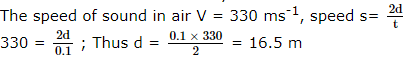Thus the minimum distance between the listener and the reflecting surface to hear the echo in air is 16.5 m (when the velocity of sound through air is 330 ms-1)

JEE Main Mock - 11 (Previous Pattern) - Question 87

For an adiabatic expansion of a perfect monoatomic gas, when volume increases by 24%, what is the percentage decrease in pressure?

JEE Main Mock - 11 (Previous Pattern) - Question 88

A water fall is 84 meters high. If half of the potential energy of the falling water gets converted to heat, the rise in temperature of water will be

JEE Main Mock - 11 (Previous Pattern) - Question 89

If a star is moving towards earth, then the lines are shifted towards

JEE Main Mock - 11 (Previous Pattern) - Question 90

The position of a particle at time 't' is given by the equation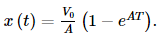. Where V0 is constant and A > 0. Dimensions of V0 and A respectively are

## Mock Test Series for JEE Main & Advanced

2 videos|324 docs|160 tests
 Use Code STAYHOME200 and get INR 200 additional OFF Use Coupon Code
Information about JEE Main Mock - 11 (Previous Pattern) Page
In this test you can find the Exam questions for JEE Main Mock - 11 (Previous Pattern) solved & explained in the simplest way possible. Besides giving Questions and answers for JEE Main Mock - 11 (Previous Pattern), EduRev gives you an ample number of Online tests for practice

## Mock Test Series for JEE Main & Advanced

2 videos|324 docs|160 tests